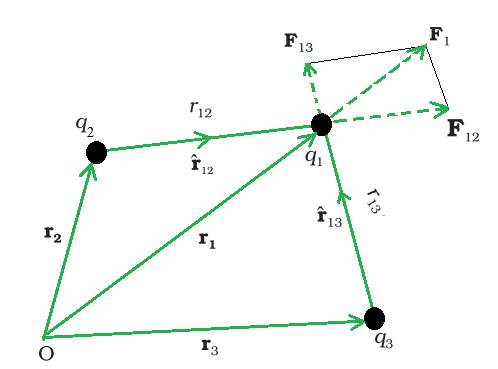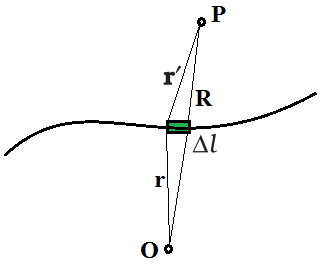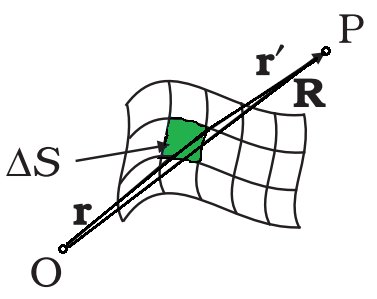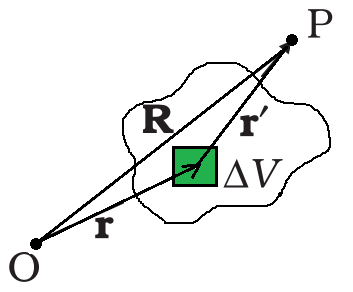Related Articles

# Superposition Principle and Continuous Charge Distribution

• Last Updated : 05 Jul, 2021

Electric charge is a fundamental feature of matter that regulates how elementary particles are impacted by an electric or magnetic field. Positive and negative electric charge exists in discrete natural units and cannot be manufactured or destroyed. There are two sorts of electric charges: positive and negative. When two items with an overabundance of one type of charge are relatively close to each other, they repel each other. When two things with excess opposing charges, one positively charged and the other negatively charged, are in proximity, they attract each other.

Electric charge is a property shared by many fundamental, or subatomic, particles of matter. Electrons, for example, have a negative charge while protons have a positive charge. Neutrons, on the other hand, have no charge. Experiments have discovered that the negative charge of each electron has the same magnitude as the positive charge of each proton. A charge is measured in natural units, which are equivalent to the charge of an electron or proton, which is a fundamental physical constant.

### Superposition Principle

The Superposition Principle allows us to determine the overall force exerted on a given charge by any number of point charges. Every charged particle in the universe produces an electric field in the surrounding space. The electric field produced by a charge is unaffected by the presence or absence of additional charges. Coulomb’s law can be used to compute the electric field created. The principle of superposition permits two or more electric fields to be combined.

According to the concept of superposition, every charge in space forms an electric field at a place irrespective of the presence of other charges in that medium. The resulting electric field is a vector sum of the constituent charges’ electric fields.

The net flux, net field, and net potential energy of the system are computed using the superposition concept.

Principle of Superposition in Electrostatics used to Calculate Force Between Multiple Charges

Consider a system in a vacuum with n motionless that is stationary charges q1, q2, and q3. It has been proven experimentally that the vector sum of all the forces on a charge due to a number of other charges, taken one at a time, is the vector sum of all the forces on that charge owing to the other charges. Due to the presence of other charges, the separate forces remain unaffected. This is known as the superposition principle.A system of three charges.

The force on one charge, say q1, due to two other charges, q2 and q3, may be determined By conducting a vector addition of the forces due to each of these charges. As a result, if F12 denotes the force exerted on q1 as a result of q2,Similarly, F13 denotes the force exerted on q1 as a result of q3, which again is the Coulomb force on q1 due to q3 even though another charge q2 is present.Thus, the total force F1 on q1 due to the two charges q2 and q3 can be expressed as,where

•andare the unit vectors along the direction of q1 and q2.
• o is the permittivity constant for the medium in which the charges are placed in.
• r12 and r13 are the distances between the charges.

### Continuous Charge Distribution

Dealt with discrete charge combinations involves q1, q2,…, qn. The mathematical treatment is easier and does not require calculus, which is one of the reasons why we limited ourselves to discrete charges. However, working with discrete charges is impracticable for many reasons, and we must instead work with continuous charge distributions. All charges are tightly bonded together with very little space between them in continuous charge distribution.

Charges can be distributed in three ways, including:

• Linear charge distribution
• Surface charge distribution
• Volume charge distribution

### Linear Charge DensityLinear Charge Density

When charges are dispersed equally along a length, such as around the circumference of a circle or along a straight wire, this is known as linear charge distribution. The linear charge distribution is symbolized by the symbol λ. The linear charge density λ of a wire is defined bywhere, ∆l is on the macroscopic scale, a small line element of wire, yet it contains a significant number of microscopic charged elements and ∆Q is the charge contained in that line element. The units for λ are C/m.

### Surface Charge DensitySurface Charge Density

It is impractical to characterize the charge distribution on the surface of a charged conductor in terms of the positions of the tiny charged elements. It is more practical to consider an area element S on the conductor’s surface (which is small on a macroscopic scale but large enough to contain numerous electrons) and specify the charge Q on that element. A surface charge density σ at the area element byThe surface charge density σ is a continuous function. The surface charge density as stated overlooks charge quantification and charge distribution discontinuities at the microscopic level. , which is a smoothed out average of the microscopic charge density across an area element ∆S, which is huge microscopically but small macroscopically, reflects macroscopic surface charge density. The unit of surface charge density σ is C/m2.

### Volume charge densityVolume charge density

Similarly, when a charge is spread uniformly over a volume then it is called volume charge distribution ρ, such as inside a sphere or a cylinder. The volume charge density ρ (also known as charge density) is defined.where ∆Q denotes the charge in the macroscopically small volume element ∆V, which contains a high number of microscopic charged constituents. The unit of volume charge density ρ is C/m3.

### Coulomb’s Law

Coulomb’s law is a mathematical formula that describes the force between two point charges. When the size of charged bodies is substantially smaller than the separation between them, then the size is not considered or can be ignored. The charged bodies can be considered as point charges.

According to Coulomb’s law, the force of attraction or repulsion between two charged things is directly proportional to the product of their charges and inversely proportional to the square of the distance between them. It acts along the line that connects the two charges that are regarded to be point charges.

Coulomb studied the force between two point charges and found that it is inversely proportional to the square of the distance between them, directly proportional to the product of their magnitudes, and acting in a line that connects them.

The amount of the force (F) between two point charges q1 and q2 separated by a distance r in a vacuum is given by,

• F ∝ q1q2 and
• F ∝ 1/r2

Therefore,

F ∝ q1q2 / r2

or

F = k q1q2 / r2

where k is proportionality constant and equals 1/4πε0.

The symbol ε0 is called epsilon not, and it signifies the permittivity of a vacuum.

The value of k is 9 × 109 Nm2/ C2. The S.I unit value of ε0 is 8.854 × 10-12 C2 N-1 m-2.

### Sample Questions

Problem 1: A circular annulus of inner radius r and outer radius R has a uniform charge density a. What will be the total charge on the annulus?

Solution:

The total surface area of the annulus is π×(R2-r2)

The surface charge density is the amount of charge stored on the unit surface area.

The surface charge density is a.

Therefore, total charge on the annulus is π×a×(R2– r2).

Problem 2: What is the dimension of linear charge density?

Solution:

The expression for the Linear charge density λ=(Amount of charge / Total length).

The dimension of electric charge is [I T] and the dimension of length is [L].

Therefore, the dimension of linear-charge-density = [I T L-1].

Problem 3: A charge is distributed along an infinite curved line in space with linear charge distribution λ. What will be the amount of force on a point charge q kept at a certain distance from the line?

Solution:

Let the point charge is situated at a distance r from a small part dl on the line.

The charge stored in stat small part is λ.dl.

The force due to that small part will be directed towards the unit vectorTherefore, force on that charge due to the entire linear charge distribution can be written as:Problem 4: A solid nonconducting sphere of radius 1 m carries a total charge of 10 C which is uniformly distributed throughout the sphere. Determine the charge density of the sphere.

Solution:

The volume of the sphere = (4/3)πr3.

where r is the radius of the sphere.

Therefore, the charge density, ρ= total charge/[(4/3)πr3].

Now substituting the values,

ρ = 10/[(4/3)πr3]

= 2.38 C/m3.

But if the sphere is conducting, we have to consider the surface charge density.

Problem 5: What is linear charge distribution?

Solution:

When charges are dispersed equally along a length, such as around the circumference of a circle or along a straight wire, this is known as linear charge distribution. The linear charge distribution is symbolized by the symbol λ. The linear charge density λ of a wire is defined bywhere, ∆l is on the macroscopic scale, a small line element of wire, yet it contains a significant number of microscopic charged elements and ∆Q is the charge contained in that line element.

The units for λ are C/m.

Attention reader! All those who say programming isn’t for kids, just haven’t met the right mentors yet. Join the  Demo Class for First Step to Coding Course, specifically designed for students of class 8 to 12.

The students will get to learn more about the world of programming in these free classes which will definitely help them in making a wise career choice in the future.

My Personal Notes arrow_drop_up